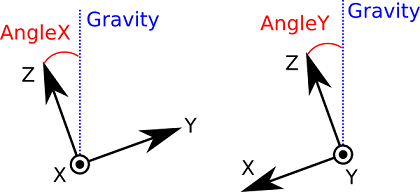Inertial unit¶

Specification¶

Pepper is equipped with an Inertial unit, made of:

• a 3-axis gyrometers with an angular speed of ~500°/s,
• a 3-axis accelerometer with an acceleration of ~2g.

Location¶

See the URDF files.

Name
AccelerometerBase_sensor
GyrometerBase_sensor

Output data¶

The output data enables an estimation of the base speed and attitude (Yaw, Pitch, Roll).

Compute Base Angle Algorithm

An SoftBank Robotics algorithm computing the base Angle from accelerometers and gyrometers is implemented inside the inertial board.

This algorithm used the good proprieties of each sensor: accelerometer is the only absolute reference and give the good base angle in static mode. When some motion is detected, the output angle is computed with gyrometers which have a good behavior in dynamic. However, integration of gyrometers creates a bias of the compute angle, so in dynamic mode, a fusion of compute angle from accelerometer and gyrometer is done to reduce this bias.Definition of AngleX and AngleY. [x, y, z] is the reference frame attached to base.

To access values by using ALMemory key names, see: Inertial Sensor.

Glossary Site map Index Support Contact Legal Notices